# NPLQCDNuclear Physics with Lattice QCD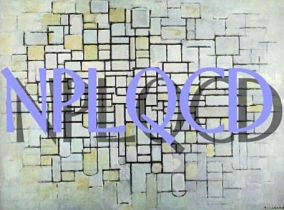Quantum Chromodynamics (QCD) is the underlying theory governing the interaction between quarks and gluons, the strong force, and therefore, responsible for all the states of matter in the Universe. Analytical solutions of QCD in the low energy regime cannot be obtained due to the complexity of the quark-gluon dynamics. The only known non-perturbative method that systematically implements QCD from first principles is its formulation on a discretized space-time, lattice QCD. This numerical simulation of the theory consists in a Monte Carlo evaluation of a functional integral. Our goal is to extract information on hadronic interactions, relevant to nuclear processes, through lattice QCD, using the enormous computing capabilities that the most modern supercomputers offer us, specially on those sectors where experiments are difficult to perform.

### Recent results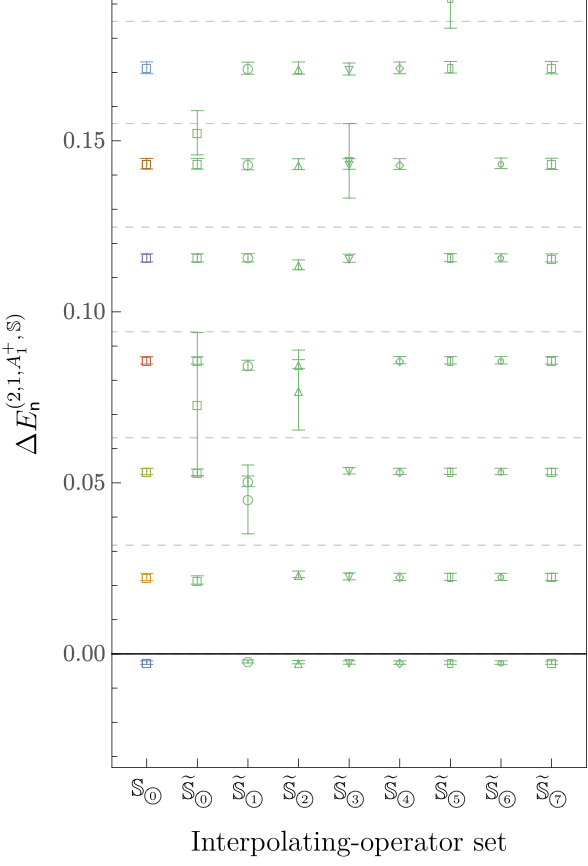A variational study of two-nucleon systems with lattice QCD
arXiv:2108.10835 [hep-lat]

The low-energy spectrum and scattering of two-nucleon systems are studied with lattice quantum chromodynamics using a variational approach. A wide range of interpolating operators are used: dibaryon operators built from products of plane-wave nucleons, hexaquark operators built from six localized quarks, and quasi-local operators inspired by two-nucleon bound-state wavefunctions in low-energy effective theories. Sparsening techniques are used to compute the timeslice-to-all quark propagators required to form correlation-function matrices using products of these operators. Projection of these matrices onto irreducible representations of the cubic group, including spin-orbit coupling, is detailed. Variational methods are applied to constrain the low-energy spectra of two-nucleon systems in a single finite volume with quark masses corresponding to a pion mass of 806 MeV. Results for S- and D-wave phase shifts in the isospin singlet and triplet channels are obtained under the assumption that partial-wave mixing is negligible. Tests of interpolating-operator dependence are used to investigate the reliability of the energy spectra obtained and highlight both the strengths and weaknesses of variational methods. These studies and comparisons to previous studies using the same gauge-field ensemble demonstrate that interpolating-operator dependence can lead to significant effects on the two-nucleon energy spectra obtained using both variational and non-variational methods, including missing energy levels and other discrepancies, as shown in the figure for the dinucleon case. While this study is inconclusive regarding the presence of two-nucleon bound states at this quark mass, it provides robust upper bounds on two-nucleon energy levels that can be improved in future calculations using additional interpolating operators and is therefore a step toward reliable nuclear spectroscopy from the underlying Standard Model of particle physics.The axial charge of the triton from lattice QCD
Phys. Rev. D 103, 074511 (2021)

The axial charge of the triton is investigated using lattice quantum chromodynamics (QCD). Calculations are performed using three ensembles of gauge field configurations generated with quark masses corresponding to a pion mass of 450 MeV. Finite-volume energy levels for the triton, as well as for the deuteron and diproton systems, are extracted from analysis of correlation functions computed on these ensembles, and the corresponding energies are extrapolated to infinite volume using finite-volume pionless effective field theory (FVEFT). It is found with high likelihood that there is a compact bound state with the quantum numbers of the triton at these quark masses. The axial current matrix elements are computed using background field techniques on one of the ensembles and FVEFT is again used to determine the axial charge of the proton and triton. A simple quark mass extrapolation of these results and earlier calculations at heavier quark masses leads to a value of the ratio of the triton to proton axial charges at the physical quark masses of $$g^{^3\text{H}}_A/g^p_A=0.91^{+0.07}_{−0.09}$$. This result is consistent with the ratio determined from experiment and prefers values less than unity (in which case the triton axial charge would be unmodified from that of the proton), thereby demonstrating that QCD can explain the modification of the axial charge of the triton.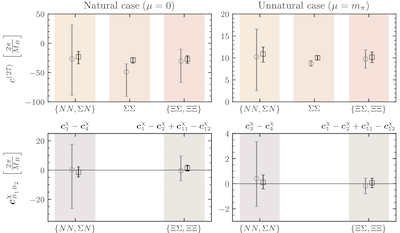The present paper presents a study of the lowest-lying spectra of several two-octet baryon systems with strangeness ranging from 0 to −4 with unphysical values of the light-quark masses. Their scattering parameters and binding momenta, determined through the use of Lüscher’s formalism, enabled to put constraints on the LO and NLO interactions of a pionless EFT, both for the SU(3) flavor-symmetric and symmetry-breaking interactions. The observation of an approximate SU(3) flavor symmetry led to an investigation of the large-$$N_c$$ predictions, and it was found that the s-wave interactions at LO exhibit an SU(6) spin-flavor symmetry. The extracted values of the coefficients suggest a remnant of the approximate accidental SU(16) symmetry observed in the SU(3) flavor-symmetric study at $$m_{\pi}\sim 806$$ MeV. The figure shows the LO SU(3) symmetric coefficient $$c^{(27)}$$ on the upper panels extracted from the spin-singlet states, and the NLO SU(3) symmetry-breaking coefficients $$c_{B_1 B_2}^{\chi}$$ on the lower panels for the same states, using the scattering parameters fixed from effective range expansion fits (gray circles) or from the binding momenta (back squares).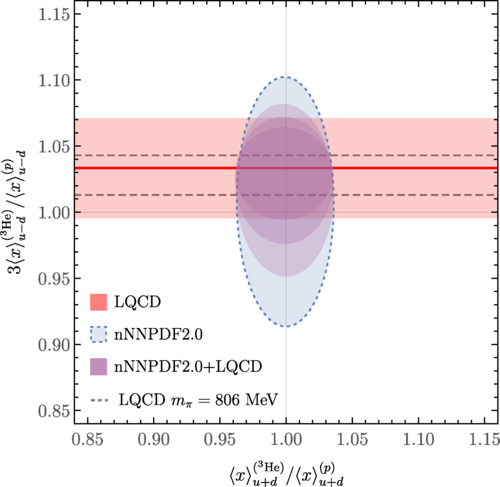Lattice QCD constraints on the parton distribution functions of 3He
Phys. Rev. Lett. 126, 202001 (2021)

In this work, the isovector momentum fractions of the proton, diproton and 3He systems have been determined using LQCD at an unphysical SU(3)-symmetric values for the quark masses corresponding to $$m_{\pi}$$ = 806 MeV. The isovector nuclear momentum fractions were calculated precisely and found to be similar to that of the proton. In particular, the ratios $$\langle x \rangle^{(pp)}_{u-d}/\langle x \rangle^{(p)}_{u-d}$$ = 1.010(14) and $$\langle x \rangle^{(^3\text{He})}_{u-d}/\langle x \rangle^{(p)}_{u-d}$$ = 1.029(15) were determined and nuclear EFT arguments were used to connect the $$^3$$He result to global analyses of nuclear PDFs, providing important constraints on the flavor decomposition of nuclear PDFs that are complementary to those obtained from experiment. The figure shows the ratio of the isovector momentum fracitons of 3He and proton determined in this work compared to constraints on the isovector and isoscalar momentum fraction ratios from the nNNPDF2.0 global analysis before and after the LQCD constraint is imposed.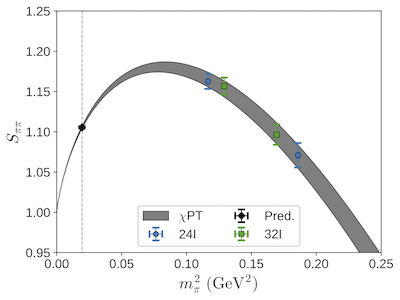In this work, a novel and general lattice QCD framework for computing four-point functions describing long-distance neutrinoless double beta decay amplitudes mediated by a light Majorana neutrino has been presented and used to compute the amplitude for the unphysical $$\pi^-\rightarrow\pi^+ e^- e^-$$ transition with pions at rest. Data for a series of lattice ensembles with pion masses in the range 300 MeV $$\lesssim m_{\pi} \lesssim$$ 430 MeV was fit to the next-to-leading-order chiral perturbation theory amplitude for this decay, and the fit was used to predict the corresponding matrix element in the physical mass, infinite volume, and continuum limit with sub-percent total uncertainty, as well as to determine the $$\chi$$PT low energy constant $$g^{\pi\pi}_{\nu}$$ with $$\approx$$ 5% uncertainty. The results were found to be consistent with other estimates of these quantities in the literature. The figures show the chiral (top left), continuum (top right) and infinite volume (bottom) extrapolation of the lattice data. The vertical dashed line in the upper left plot corresponds to the physical $$m_{\pi^-}$$ = 139.5702(4) MeV.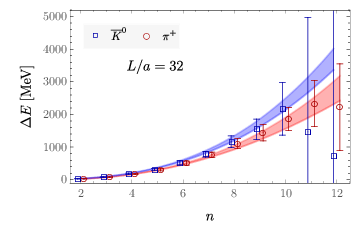Charged multi-hadron systems in lattice QCD+QED
Phys. Rev. D 103, 054504 (2021)

In this joint work with the QCDSF collaboration, lattice QCD+QEDL has been used to study systems of up to 12 charged or neutral mesons as well as systems of one, two, and three nucleons. Analysis of the finite-volume energy levels for multi-meson systems using non-relativistic EFT has allowed the extraction of the $$\pi^+ \pi^+$$ and $$K^0 K^0$$ scattering lengths as well as the 3$$\pi^+$$ and 3$$K^0$$ interaction parameters. The QED effects on multi-meson systems determined from LQCD+QEDL are well-described by NRQEDL results that incorporate short-range two- and three-body contact interactions as well as perturbative Coulomb photon exchange. The range of values for the EM fine structure constant $$\alpha$$, the quark masses and lattice size L are explored to see where Coulomb effects can be perturbative. Figure shows finite volume energy shifts for $$n$$-pions (red circles), $$n$$-kaons (blue squares), and the predictions (fitted bands) obtained from N3LO NRQEDL.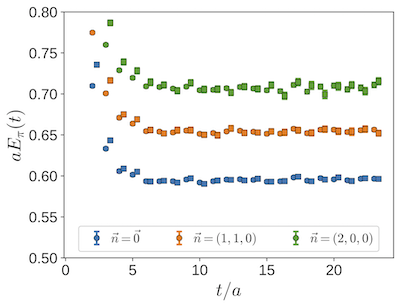Sparsening Algorithm for Multi-Hadron Lattice QCD Correlation Functions
Phys. Rev. D 104, 034502 (2021)

This work explores a propagator sparsening algorithm for forming correlation functions describing multi-hadron systems, such as light nuclei, with reduced computational cost. Using a relatively simple prescription for sparsening is sufficient to preserve the ground state energies and uncertainties extracted from a lattice QCD simulation. Also, this sparsening procedure alters the couplings to excited states observed at early Euclidean times for single- and multi-hadron correlation functions; however, a simple modification of the sparsified correlation functions can efficiently remove these modified excited state effects, if desired. The sparsening techniques that are presented here enable $$\mathcal{O}$$(10−100) fold speedups in the contraction stage of lattice calculations of nuclear physics, and this factor will further increase as the continuum limit is approached. Figure shows the of the pion with different lattice momenta $$\vec{n}$$. Circles (squares) denote data computed from full (sparsened) two-point correlation functions.Nuclear modification of scalar, axial and tensor charges from lattice QCD
Phys. Rev. Lett. 120, 152002 (2018)

In this Letter, a study of the scalar, axial and tensor charges of the nucleon and light nuclei up to atomic number A = 3 is presented at a single set of unphysical values of the quark masses corresponding to a pion mass of $$m_{\pi}$$ ∼ 806 MeV and at one lattice spacing. The present study reveals that nuclear effects in the scalar charges are large compared to those in the axial and tensor charges for light nuclei. If this hierarchy persists at the physical values of the quark masses and for larger nuclei, nuclear effects in the scalar charges will be of critical importance in direct searches for dark matter in experiments and in the refinement of mean-field models of large nuclei and fission processes. Moreover, while disconnected diagrams are found to give negligible contributions to the nuclear axial and tensor charges, they make significant contributions to the scalar charges of nuclei. The figure shows the deviation from naive expectation of the ratios of charges in the deuteron (circles), diproton (diamonds) and 3He (squares) to those in the proton. The panels show the scalar (top), axial (middle) and tensor (bottom) current results and the columns within the panels display results for the different flavour structures of the currents, as indicated at the top of the figure. In each case, the statistical and systematic uncertainties have been combined in quadrature. The points exactly at zero are constrained to vanish by spin and/or isospin symmetry, while ratios are not given for the strange quark axial and tensor charges as both the numerators and denominator are consistent with zero.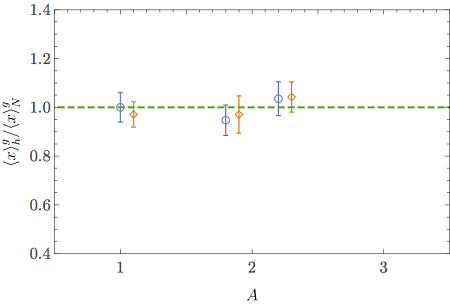First lattice QCD study of the gluonic structure of light nuclei
Phys. Rev. D 96, 094512 (2017)

In this work, the gluonic structure of light nuclei is investigated for the first time. The first moments of the unpolarised gluon densities in spin-averaged nuclei (the gluon momentum fractions) and of the gluon transversity are studied in nuclear states of atomic number A ≤ 3 at two different sets of quark masses corresponding to pion masses of $$m_{\pi}$$ ∼ 450 and 806 MeV. Nuclear modification of this quantity defines a gluonic analogue of the EMC effect and is constrained to be less than ∼ 10% in these nuclei. This is consistent with expectations from phenomenological quark distributions and the momentum sum rule. This study shows that these quantities can be accessed in LQCD, and includes estimates of the computational requirements to perform calculations that more directly impact phenomenology by resolving a gluonic analogue of the EMC effect and the first moment of the gluon transversity at lighter values of the quark masses. The plots show the ratios of the gluon momentum fraction in the nucleon and light nuclei $$h = \{N, d, nn, ^3 \hskip -0.03in\text{He}\}$$ from left to right, to the central value of this quantity in the nucleon with SP source-sink smearing. The top and bottom panels show results for the ensemble with $$m_{\pi}$$ ∼ 450 MeV and $$m_{\pi}$$ ∼ 806 MeV, respectively. The blue circles and orange diamonds denote results obtained using SP and SS source-sink smearing combinations. The green dashed line is at 1, shown to guide the eye.Baryon-Baryon Interactions and Spin-Flavor Symmetry from Lattice Quantum Chromodynamics
Phys. Rev. D 96, 114510 (2017)

This paper presents the results of a lattice QCD study of low-energy S-wave scattering amplitudes of two octet baryons at an SU(3) flavor-symmetric point, with a focus on underlying symmetry structures that are expected to emerge in the large-$$N_c$$ limit of QCD. At a pion mass of ∼ 806 MeV, S-wave interactions between two baryons in the $$27$$, $$10$$ and $$8_A$$ irreps (e.g., $$NN\; ( ^1 S_0)$$ , $$NN\; ( ^3 S_1)$$ and $$\frac{1}{\sqrt{2}}(\Xi^0 n + \Xi^− p)\; ( ^3S_1)$$, respectively) are found to induce bound states, consistent with our previous analyses of the same correlation functions. The presence of a bound state in the $$10$$ irrep (e.g. $$\Sigma^+ p \; ( ^3S_1)$$) is not statistically significant. The scattering lengths and effective ranges in the four channels have been extracted, and suggest that all of these systems have unnaturally large scattering lengths, with $$|r/a|$$ ∼ 0.5. If this feature is found to persist in the hyperon channels at the physical values of the quark masses, its phenomenological consequences would be interesting to explore. The values of the leading-order low-energy scattering parameters in the irreducible representations of SU(3) are consistent with an approximate SU(6) spin-flavor symmetry in the nuclear and hypernuclear forces that is predicted in the large-$$N_c$$ limit of QCD. The two distinct SU(6)-invariant interactions between two baryons are constrained at this value of the quark masses, and their values indicate an approximate accidental SU(16) symmetry. The plots show a comparison of the values of the inverse scattering length, $$a^{−1}$$, effective range, $$r$$, the first shape parameter, $$P$$ , and the ratio $$r/a$$, obtained from fits to the two-parameter ($$N_{\text{ERE}}$$ = 2) and three-parameter ($$N_{\text{ERE}}$$ = 3) effective range expansion, in channels belonging to the four different irreps.Double-$$\beta$$ Decay Matrix Elements from Lattice Quantum Chromodynamics
Phys. Rev. D 96, 054505 (2017)

Expanding on the results presented in the previous Letter, this paper explains the recently-developed fixed-order background-field approach to cleanly isolate matrix elements corresponding to a fixed number of insertions of the isovector axial current.Further details of this method, along with the associated analysis techniques used to extract the $$nn \rightarrow pp$$ transition matrix element, are presented. Second-order weak processes are discussed in the dibaryon formulation of pionless EFT whose finite-volume Euclidean-space correlation functions are constructed and matched to the LQCD correlation functions, allowing a determination of the leading two-nucleon second-order weak coupling.In conjunction with many-body methods, these couplings can be used to predict $$\beta \beta$$-decay rates of nuclei at these quark masses. The plots show the bare short-distance contribution to the $$nn \rightarrow pp$$ matrix element at late times (top) and the sum of long and short-distance contribut to the matrix element (bottom). Orange (blue) dots correspond to the smeared-smeared (smeared-point) results.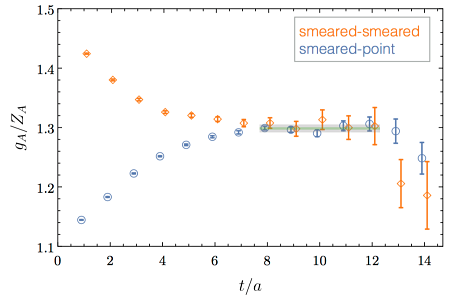Proton-Proton Fusion and Tritium $$\beta$$-Decay from Lattice Quantum Chromodynamics
Phys. Rev. Lett. 119, 062002 (2017)

In this Letter, the nuclear matrix element determining the $$p p \rightarrow d e^+ \nu_e$$ fusion cross section and the Gamow-Teller matrix element contributing to tritium $$\beta$$-decay are calculated with lattice QCD for the first time. Although we use unphysically large values of the light quark masses ($$m_{\pi}\sim$$ 806 MeV) and the effects of isospin-breaking and electromagnetism are neglected, all the results obtained (with very high precsion) are consistent with experimental values or phenomenological estimates. All in all, this work demonstrates that weak transition amplitudes in few-nucleon systems can be studied directly from the fundamental quark and gluon degrees of freedom and opens the way for subsequent investigations of many important quantities in nuclear physics. The plot shows the unrenormalized isovector axial charge of the proton for different correlation functions. Including the renormalization factor yields an axial charge of $$g_A$$ = 1.13(2)(7), which is consistent with previous determinations at this pion mass.

Featured in Physics: Strong Force Calculations for Weak Force Reactions

and selected as an Editor's suggestionOctet Baryon Magnetic Moments from Lattice QCD: Approaching Experiment from a Three-Flavor Symmetric Point
Phys. Rev. D 95, 114513 (2017)

Magnetic moments of the octet baryons are computed using lattice QCD in background magnetic fields with pion masses of $$m_{\pi}\sim$$ 450 and 800 MeV. As shown in the figure, the anomaouls magnetic moments are found to exhibit only mild pion-mass dependence when expressed in terms of appropriately chosen magneton units - the natural baryon magneton, $$\texttt{nBM}$$ (the mass of each baryon computed with LQCD is used to define its magnetic moment), and also they reveal a pattern linked to the constituent quark model. We also study relations expected to hold in the large-$$N_c$$ limit of QCD and the magnetically coupled $$\Sigma^0-\Lambda$$ system.Unitary Limit of Two-Nucleon Interactions in Strong Magnetic Fields
Phys. Rev. Lett. 116, 112301 (2016)

In this work, lattice QCD calculations of the energies of one and two nucleons systems are performed at pion masses of $$m_{\pi}\sim$$ 450 and 806 MeV in uniform, time-independent magnetic fields to determine the response of these hadronic systems. Having found significant changes in the binding energy of two-nucleon systems immersed in strong magnetic fields at two values of unphysical quark masses, it is conceivable that similar modifications occur in nature. The figure shows the response of the binding energy of one the systems studied, the dineutron, to different magnetic fields $$|e\mathbf{B}|=6\pi|\tilde{n}|/(aL)^2$$ and different pion masses. The horizontal red-shaded bands show the breakup threshold for the dineutron, above which the ground state of the system would be two neutrons in the continuum in the $$^1 \hskip -0.03in S_0$$ channel.Two Nucleon Systems at $$m_{\pi}\sim$$ 450 MeV from Lattice QCD
Phys. Rev. D 92, 114512 (2015)

In this paper, nucleon-nucleon systems are studied with lattice QCD at a pion mass of $$m_{\pi}\sim$$ 450 MeV in three spatial volumes, $$L=$$ 2.8 fm, 3.7 fm and 5.6 fm, using $$n_f=$$ 2 + 1 flavors of light quarks. The study includes the $$^3 \hskip -0.025in S_1 - ^3 \hskip -0.04in D_1$$ coupled channel, the deuteron, and the $$^1 \hskip -0.03in S_0$$ channel, the dineutron. Both are found to be bound at this pion mass, consistent with expectations based upon previous calculations.In both channels, the phase shifts are determined at three energies that lie within the radius of convergence of the effective range expansion,allowing for constraints to be placed on the inverse scattering lengths and effective ranges. These are then used to extract low energy counterterms from nuclear effective field theories. Finally, the violation of the Gell-Mann–Okubo mass relation is analyzed. In the plots, we show the pion mass dependence of the deuteron (left) and dineutron (right) binding energies calculated with LQCD by different collaborations (NPLQCD and Yamazaki et al.).

Selected as an Editor's suggestionThe Magnetic Structure of Light Nuclei from Lattice QCD
Phys. Rev. D 92, 114502 (2015)

The magnetic moments and magnetic polarizabilities of the nucleons and of light nuclei with A ≤ 4, along with the cross-section for the M1 transition $$np\rightarrow d\gamma$$, have been calculated at the flavor SU(3)-symmetric point where the pion mass is $$m_{\pi}\sim$$ 806 MeV using LQCD in the presence of background magnetic fields. These magnetic properties are extracted from nucleon and nuclear energies in six uniform magnetic fields of varying strengths. The magnetic moments were presented in this Letter, and the cross-section for $$np\rightarrow d\gamma$$ was combined with an analogous result at $$m_{\pi}\sim$$ 450 MeV to extrapolate to the physical point in this Letter. The figure shows a summary of the magnetic polarizabilities in physical units.Ab initio calculation of the $$np\rightarrow d\gamma$$ radiative capture process
Phys. Rev. Lett. 115, 132001 (2015)

In this work, lattice QCD calculations have been used to determine the short-distance two-nucleon interactions with the electromagnetic field that make significant contributions to the low-energy cross-sections for $$np\rightarrow d\gamma$$ and $$\gamma^{(*)}d\rightarrow np$$. Calculations of neutron-proton energy levels in multiple background magnetic fields are performed at two values of the quark masses, corresponding to pion masses of $$m_{\pi}\sim$$ 450 and 806 MeV, and are combined with pionless nuclear effective field theory to determine these low-energy inelastic processes. Extrapolating to the physical pion mass, a cross section of $$\sigma^{\text{lqcd}}(np\rightarrow d\gamma)$$ is consistent with the experimental value. In the plot, we show the extrapolation of the coefficient $$\overline{L}_1$$, the only quantity that is not determined by kinematics, single-nucleon properties or scattering parameters.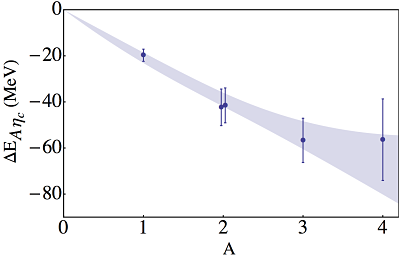Quarkonium-Nucleus Bound States from Lattice QCD
Phys. Rev. D 91, 114503 (2015)

In this paper, we present lattice QCD calculations of the interactions of strange and charm quarkonia with light nuclei. In the plot, we represent the binding energies for the charm quarkonia as a function of the atomic number. Both the strangeonium-nucleus and charmonium-nucleus systems are found to be relatively deeply bound when the masses of the three light quarks are set equal to that of the physical strange quark. Extrapolation of these results to the physical light-quark masses suggests that the binding energy of charmonium to nuclear matter is $$B_{\text{phys}}^{\text{NM}}\lesssim$$ 40 MeV.Magnetic moments of light nuclei from lattice quantum chromodynamics
Phys. Rev. Lett. 113, 252001 (2014)

We recently demonstrated for the first time that Quantum Chromodynamics (QCD) can be used to calculate the structure of nuclei from first principles. This study performed exploratory lattice QCD calculations of the magnetic moments of light nuclear systems, at the SU(3) flavor symmetric point, corresponding to a pion mass of 800 MeV. When presented in terms of the natural nuclear magneton at the corresponding quark masses, the extracted magnetic moments were seen to be in close agreement with those of nature. Additionally, the magnetic moment of $$^3\text{He}$$ was found to be close to that of the neutron, and that of the triton was close to that of the proton, in agreement with the expectations of the phenomenological shell-model, and therefore suggesting that this model structure is a robust feature of nuclei even away from the physical quark masses.Light Nuclei and Hypernuclei from Quantum Chromodynamics in the Limit of SU(3) Flavor Symmetry
Phys. Rev. D 87, 034506 (2013)

In this work we have calculated the binding energies of a range of nuclei and hypernuclei with atomic number A up to 4 and strangeness 0, -1 and -2. The calculations were performed in the limit of flavor-SU(3) symmetry, at the physical strange quark mass and in the absence of electromagnetic interactions. The study includes the deuteron, di-neutron, H-dibaryon, $$^3\text{He}$$, $$_{\Lambda}^3\text{He}$$, $$_{\Lambda}^4\text{He}$$, and $$^{\phantom{\Lambda}4}_{\Lambda\Lambda}\text{He}$$. The nuclear states are extracted from lattice QCD calculations performed with $$n_f=$$ 3 dynamical light quarks using an isotropic clover discretization of the quark-action in three lattice volumes of spatial extent L ~ 3.4 fm, 4.5 fm and 6.7 fm, and with a single lattice spacing b ~ 0.145 fm. In the figure, we show a compilation of the nuclear energy levels, with spin and parity as determined in this work.

### Who we are

From left to right:
Silas R. Beane (U of Washington), Emmanuel Chang,
Zohreh Davoudi (U of Maryland), William Detmold (MIT), Marc Illa (U of Washington),
William Jay (MIT), Kostas Orginos (William & Mary and TJNAF),
Assumpta Parreño (U de Barcelona), Martin J. Savage (U of Washington),
Phiala Shanahan (MIT), Brian Tiburzi (City College of New York),
Michael Wagman (Fermilab), Frank Winter (TJNAF).